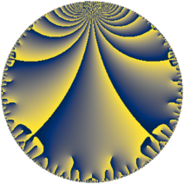# Properties

 Label 27.7.dLevel $27$ Weight $7$ Character orbit 27.d Rep. character $\chi_{27}(8,\cdot)$ Character field $\Q(\zeta_{6})$ Dimension $10$ Newform subspaces $1$ Sturm bound $21$ Trace bound $0$

# Related objects

## Defining parameters

 Level: $$N$$ $$=$$ $$27 = 3^{3}$$ Weight: $$k$$ $$=$$ $$7$$ Character orbit: $$[\chi]$$ $$=$$ 27.d (of order $$6$$ and degree $$2$$) Character conductor: $$\operatorname{cond}(\chi)$$ $$=$$ $$9$$ Character field: $$\Q(\zeta_{6})$$ Newform subspaces: $$1$$ Sturm bound: $$21$$ Trace bound: $$0$$

## Dimensions

The following table gives the dimensions of various subspaces of $$M_{7}(27, [\chi])$$.

Total New Old
Modular forms 42 14 28
Cusp forms 30 10 20
Eisenstein series 12 4 8

## Trace form

 $$10 q + 3 q^{2} + 127 q^{4} + 219 q^{5} - 121 q^{7} + O(q^{10})$$ $$10 q + 3 q^{2} + 127 q^{4} + 219 q^{5} - 121 q^{7} - 132 q^{10} - 483 q^{11} - 841 q^{13} - 12174 q^{14} - 1985 q^{16} + 6176 q^{19} + 63186 q^{20} + 3471 q^{22} - 53565 q^{23} + 8452 q^{25} - 22660 q^{28} + 80679 q^{29} - 24601 q^{31} - 218295 q^{32} + 7425 q^{34} + 12764 q^{37} + 371877 q^{38} + 54150 q^{40} - 232251 q^{41} - 93271 q^{43} + 112512 q^{46} + 142887 q^{47} + 86238 q^{49} - 318459 q^{50} + 186920 q^{52} - 419982 q^{55} - 342546 q^{56} - 380658 q^{58} + 995061 q^{59} - 59305 q^{61} + 403066 q^{64} - 1642029 q^{65} + 158513 q^{67} + 1693791 q^{68} - 304788 q^{70} + 933896 q^{73} - 595182 q^{74} + 666641 q^{76} + 2198883 q^{77} + 468707 q^{79} - 2038470 q^{82} - 3008337 q^{83} - 1189944 q^{85} - 1905549 q^{86} - 349773 q^{88} - 211778 q^{91} + 973788 q^{92} + 809124 q^{94} + 2562954 q^{95} + 336029 q^{97} + O(q^{100})$$

## Decomposition of $$S_{7}^{\mathrm{new}}(27, [\chi])$$ into newform subspaces

Label Dim $A$ Field CM Traces $q$-expansion
$a_{2}$ $a_{3}$ $a_{5}$ $a_{7}$
27.7.d.a $10$ $6.211$ $$\mathbb{Q}[x]/(x^{10} - \cdots)$$ None $$3$$ $$0$$ $$219$$ $$-121$$ $$q+\beta _{1}q^{2}+(\beta _{1}+\beta _{2}-5^{2}\beta _{4}+\beta _{5})q^{4}+\cdots$$

## Decomposition of $$S_{7}^{\mathrm{old}}(27, [\chi])$$ into lower level spaces

$$S_{7}^{\mathrm{old}}(27, [\chi]) \cong$$ $$S_{7}^{\mathrm{new}}(9, [\chi])$$$$^{\oplus 2}$$